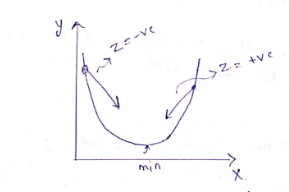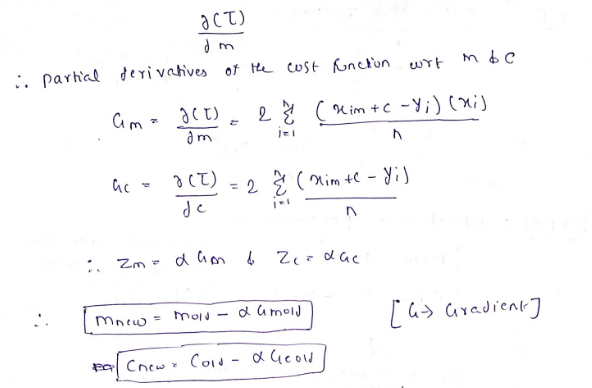top of page
• rishabhdwivedi062

# How to optimize linear regression model using gradient descent.

Updated: Oct 5, 2022

It is an optimization algorithm that works iteratively and aims to find the minimum value of a convex function with respect to a set of parameters.

You can call it a hit-and-trial method.

Let's understand it with a simple but effective example.For example, if we have a ball and we need to through it in a basket, we will try throwing it at certain angle, it may possible we can't get the goal hit, but we get a rough idea about the angle, we will try again, if fails, then again our angle will improve and so on. Finally with this hit and trial approach we will get the goal hit. The same thing works for Gradient Descent.

1) Random Initialization

Take random value of slope(m) and intercept(c). To avoid everything from scratch, you can take the slope as 0.1 and intercept as a mean of the target value.

2) Generate Prediction

Solve the linear equation using initialization value.

Y = mx+c

3) Calculating the cost

Cost calculation is the most important part of this step, we can calculate using the given formula.4) Updation of Parameter

As we know new answer is derived from the old values.Z is an unknown quantity, it can be positive or negative.

If Z is positive, then the parameter will decrease.

If Z is negative, then the parameter will increase.But how to know on which side of the minimum cost we are on and what value should we take for Z?

The solution is Partial DifferentiationThis calculation is pretty straightforward.

If the value of Alfa is High then the cost function may explode and bounce out far from the minima.

If the value of Alfa is Low then parameters will take a lot of iteration to converge to the optimal values.

We will code in the upcoming projects.